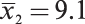## Quiz: Two-Sample t-test for Comparing Two Means

Two random samples have been selected and a 95% confidence interval for the difference in population means μ 1 – μ 2, is constructed. The results are s 1 = 2.5,, n 1 = 30, s 2 = 2.9,, n 2 = 35, and df = 29. Use this data for questions 4 and 5.